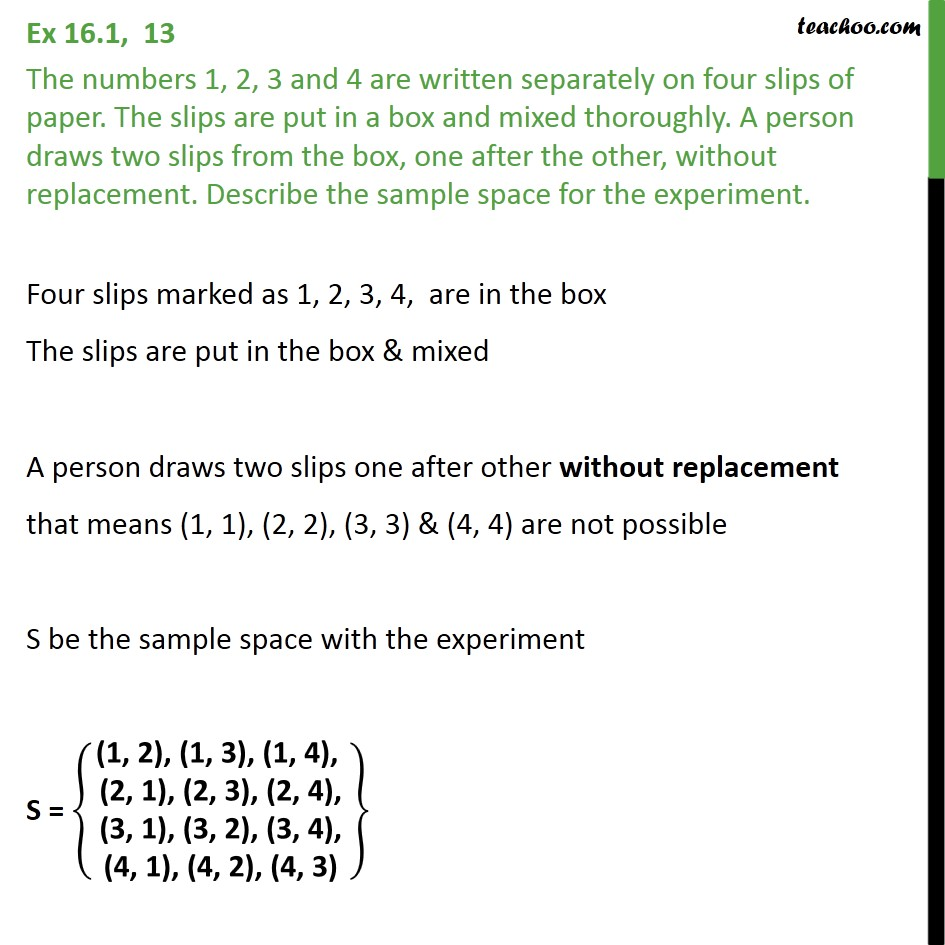1. Chapter 16 Class 11 Probability
2. Concept wise
3. Sample Space

Transcript

Ex 16.1, 13 The numbers 1, 2, 3 and 4 are written separately on four slips of paper. The slips are put in a box and mixed thoroughly. A person draws two slips from the box, one after the other, without replacement. Describe the sample space for the experiment. Four slips marked as 1, 2, 3, 4, are in the box The slips are put in the box & mixed A person draws two slips one after other without replacement that means (1, 1), (2, 2), (3, 3) & (4, 4) are not possible S be the sample space with the experiment S = (1, 2), (1, 3), (1, 4), (2, 1), (2, 3), (2, 4), (3, 1), (3, 2), (3, 4), (4, 1), (4, 2), (4, 3)

Sample Space